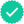Question

Expert SolutionStep 1

In natural vibrations we assume there is no friction.

Since there is no friction, total energy of the system is conserved.

Since total energy remains constant ,time derivative of total energy will be zero.

By using this condition, we can develop equation of motion for the simple pendulum.

Step 2

If the pendulum is set into vibration ,we can find total energy of the simple pendulum at some instant during its motion.

Total Energy = Kinetic Energy + Potential Energy

Step 3

We can find total energy of the simple pendulum as follows.(Refer to Figure 1).

Step 4

Since total energy remains constant in natural vibrations,

Step 5

I= mass moment of Inertia of the pendulum about point 0.

I=ML

Sustituting the value of I in Equation(1) we have:

Step 6